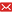UM  > Faculty of Science and Technology  > DEPARTMENT OF CIVIL AND ENVIRONMENTAL ENGINEERING
 Status 已發表Published Forced vibration analysis of functionally graded beams by the meshfree boundary-domain integral equation method Yang, Y.; Lam, C. C.; Kou, K. P. 2016-11-01 Source Publication Engineering Analysis with Boundary ElementsISSN 0955-7997 Pages 100-110 Abstract Forced vibration of two-dimensional functionally graded beams is studied in this paper by a developed meshfree boundary-domain integral equation method. Material properties of the functionally graded beams are assumed varying continuously either in longitudinal or transvers direction following the exponential function. The boundary-domain integral equations are derived by using the elastostatic fundamental solutions based on the two-dimensional elastic theory. Radial integral method (RIM) is employed to transform the domain integrals into boundary integrals. A meshfree scheme is achieved through assuming the displacements and accelerations in the domain integrals by a combination of the radial basis function and polynomials with time dependent coefficients. Wilson-θ, Houbolt as well as two kinds of damped Newmark's algorithms are applied to accomplish the time integration. The forced vibration of the functionally graded beam subjected by the harmonic loading and transient loading are investigated in detail. Numerical examples demonstrate that the four mentioned time integral schemes are all adapted well to the developed meshfree boundary-domain integral equation method for analyzing the forced vibration of homogeneous structures. For the analysis of FG structures, it is shown that the damped Newmark's algorithm can achieve more stable and accurate results. Keyword Functionally graded beams Forced vibration dynamic analysis Meshfree boundary-domain integral equation method Radial integral method (RIM) Finite element analysis Language 英語English The Source to Article PB_Publication PUB ID 25705 Document Type Journal article Collection DEPARTMENT OF CIVIL AND ENVIRONMENTAL ENGINEERING Corresponding Author Lam, C. C. Recommended CitationGB/T 7714 Yang, Y.,Lam, C. C.,Kou, K. P.. Forced vibration analysis of functionally graded beams by the meshfree boundary-domain integral equation method[J]. Engineering Analysis with Boundary Elements,2016:100-110. APA Yang, Y.,Lam, C. C.,&Kou, K. P..(2016).Forced vibration analysis of functionally graded beams by the meshfree boundary-domain integral equation method.Engineering Analysis with Boundary Elements,100-110. MLA Yang, Y.,et al."Forced vibration analysis of functionally graded beams by the meshfree boundary-domain integral equation method".Engineering Analysis with Boundary Elements (2016):100-110.
 Files in This Item: There are no files associated with this item.
 Related Services Recommend this item Bookmark Usage statistics Export to Endnote Google Scholar Similar articles in Google Scholar [Yang, Y.]'s Articles [Lam, C. C.]'s Articles [Kou, K. P.]'s Articles Baidu academic Similar articles in Baidu academic [Yang, Y.]'s Articles [Lam, C. C.]'s Articles [Kou, K. P.]'s Articles Bing Scholar Similar articles in Bing Scholar [Yang, Y.]'s Articles [Lam, C. C.]'s Articles [Kou, K. P.]'s Articles Terms of Use No data! Social Bookmark/Share